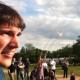author Ben GamariMon, 4 Jul 2016 12:36:44 +0000 (14:36 +0200) committer Ben GamariMon, 4 Jul 2016 21:35:25 +0000 (23:35 +0200)
Test Plan: Validate, verify fusion

Reviewers: austin, hvr

Subscribers: thomie

GHC Trac Issues: #12354

index e09d2a9..a8b6600 100644 (file)
@@ -614,26 +614,150 @@ instance Enum Word where
| x <= maxIntWord = I# (word2Int# x#)
| otherwise       = fromEnumError "Word" x

-    enumFrom n             = map integerToWordX [wordToIntegerX n .. wordToIntegerX (maxBound :: Word)]
-    enumFromTo n1 n2       = map integerToWordX [wordToIntegerX n1 .. wordToIntegerX n2]
-    enumFromThenTo n1 n2 m = map integerToWordX [wordToIntegerX n1, wordToIntegerX n2 .. wordToIntegerX m]
-    enumFromThen n1 n2     = map integerToWordX [wordToIntegerX n1, wordToIntegerX n2 .. wordToIntegerX limit]
-      where
-         limit :: Word
-         limit  | n2 >= n1  = maxBound
-                | otherwise = minBound
+    {-# INLINE enumFrom #-}
+    enumFrom (W# x#)      = eftWord x# maxWord#
+        where !(W# maxWord#) = maxBound
+        -- Blarg: technically I guess enumFrom isn't strict!
+
+    {-# INLINE enumFromTo #-}
+    enumFromTo (W# x) (W# y) = eftWord x y
+
+    {-# INLINE enumFromThen #-}
+    enumFromThen (W# x1) (W# x2) = efdWord x1 x2
+
+    {-# INLINE enumFromThenTo #-}
+    enumFromThenTo (W# x1) (W# x2) (W# y) = efdtWord x1 x2 y

maxIntWord :: Word
-- The biggest word representable as an Int
maxIntWord = W# (case maxInt of I# i -> int2Word# i)

--- For some reason integerToWord and wordToInteger (GHC.Integer.Type)
--- work over Word#
-integerToWordX :: Integer -> Word
-integerToWordX i = W# (integerToWord i)
+-----------------------------------------------------
+-- eftWord and eftWordFB deal with [a..b], which is the
+-- most common form, so we take a lot of care
+-- In particular, we have rules for deforestation
+
+{-# RULES
+"eftWord"        [~1] forall x y. eftWord x y = build (\ c n -> eftWordFB c n x y)
+"eftWordList"     eftWordFB  (:) [] = eftWord
+ #-}
+
+-- The Enum rules for Word work much the same way that they do for Int.
+-- See Note [How the Enum rules work].

-wordToIntegerX :: Word -> Integer
-wordToIntegerX (W# x#) = wordToInteger x#
+{-# NOINLINE  eftWord #-}
+eftWord :: Word# -> Word# -> [Word]
+-- [x1..x2]
+eftWord x0 y | isTrue# (x0 `gtWord#` y) = []
+             | otherwise                = go x0
+                where
+                  go x = W# x : if isTrue# (x `eqWord#` y)
+                                then []
+                                else go (x `plusWord#` 1##)
+
+{-# INLINE  eftWordFB #-}
+eftWordFB :: (Word -> r -> r) -> r -> Word# -> Word# -> r
+eftWordFB c n x0 y | isTrue# (x0 `gtWord#` y) = n
+                   | otherwise                = go x0
+                  where
+                    go x = W# x `c` if isTrue# (x `eqWord#` y)
+                                    then n
+                                    else go (x `plusWord#` 1##)
+                        -- Watch out for y=maxBound; hence ==, not >
+        -- Be very careful not to have more than one "c"
+        -- so that when eftInfFB is inlined we can inline
+        -- whatever is bound to "c"
+
+
+-----------------------------------------------------
+-- efdWord and efdtWord deal with [a,b..] and [a,b..c].
+-- The code is more complicated because of worries about Word overflow.
+
+-- See Note [How the Enum rules work]
+{-# RULES
+"efdtWord"       [~1] forall x1 x2 y.
+                     efdtWord x1 x2 y = build (\ c n -> efdtWordFB c n x1 x2 y)
+"efdtWordUpList"   efdtWordFB (:) [] = efdtWord
+ #-}
+
+efdWord :: Word# -> Word# -> [Word]
+-- [x1,x2..maxWord]
+efdWord x1 x2
+ | isTrue# (x2 `geWord#` x1) = case maxBound of W# y -> efdtWordUp x1 x2 y
+ | otherwise                 = case minBound of W# y -> efdtWordDn x1 x2 y
+
+{-# NOINLINE  efdtWord #-}
+efdtWord :: Word# -> Word# -> Word# -> [Word]
+-- [x1,x2..y]
+efdtWord x1 x2 y
+ | isTrue# (x2 `geWord#` x1) = efdtWordUp x1 x2 y
+ | otherwise                 = efdtWordDn x1 x2 y
+
+{-# INLINE  efdtWordFB #-}
+efdtWordFB :: (Word -> r -> r) -> r -> Word# -> Word# -> Word# -> r
+efdtWordFB c n x1 x2 y
+ | isTrue# (x2 `geWord#` x1) = efdtWordUpFB c n x1 x2 y
+ | otherwise                 = efdtWordDnFB c n x1 x2 y
+
+-- Requires x2 >= x1
+efdtWordUp :: Word# -> Word# -> Word# -> [Word]
+efdtWordUp x1 x2 y    -- Be careful about overflow!
+ | isTrue# (y `ltWord#` x2) = if isTrue# (y `ltWord#` x1) then [] else [W# x1]
+ | otherwise = -- Common case: x1 <= x2 <= y
+               let !delta = x2 `minusWord#` x1 -- >= 0
+                   !y' = y `minusWord#` delta  -- x1 <= y' <= y; hence y' is representable
+
+                   -- Invariant: x <= y
+                   -- Note that: z <= y' => z + delta won't overflow
+                   -- so we are guaranteed not to overflow if/when we recurse
+                   go_up x | isTrue# (x `gtWord#` y') = [W# x]
+                           | otherwise                = W# x : go_up (x `plusWord#` delta)
+               in W# x1 : go_up x2
+
+-- Requires x2 >= x1
+efdtWordUpFB :: (Word -> r -> r) -> r -> Word# -> Word# -> Word# -> r
+efdtWordUpFB c n x1 x2 y    -- Be careful about overflow!
+ | isTrue# (y `ltWord#` x2) = if isTrue# (y `ltWord#` x1) then n else W# x1 `c` n
+ | otherwise = -- Common case: x1 <= x2 <= y
+               let !delta = x2 `minusWord#` x1 -- >= 0
+                   !y' = y `minusWord#` delta  -- x1 <= y' <= y; hence y' is representable
+
+                   -- Invariant: x <= y
+                   -- Note that: z <= y' => z + delta won't overflow
+                   -- so we are guaranteed not to overflow if/when we recurse
+                   go_up x | isTrue# (x `gtWord#` y') = W# x `c` n
+                           | otherwise                = W# x `c` go_up (x `plusWord#` delta)
+               in W# x1 `c` go_up x2
+
+-- Requires x2 <= x1
+efdtWordDn :: Word# -> Word# -> Word# -> [Word]
+efdtWordDn x1 x2 y    -- Be careful about underflow!
+ | isTrue# (y `gtWord#` x2) = if isTrue# (y `gtWord#` x1) then [] else [W# x1]
+ | otherwise = -- Common case: x1 >= x2 >= y
+               let !delta = x2 `minusWord#` x1 -- <= 0
+                   !y' = y `minusWord#` delta  -- y <= y' <= x1; hence y' is representable
+
+                   -- Invariant: x >= y
+                   -- Note that: z >= y' => z + delta won't underflow
+                   -- so we are guaranteed not to underflow if/when we recurse
+                   go_dn x | isTrue# (x `ltWord#` y') = [W# x]
+                           | otherwise                = W# x : go_dn (x `plusWord#` delta)
+   in W# x1 : go_dn x2
+
+-- Requires x2 <= x1
+efdtWordDnFB :: (Word -> r -> r) -> r -> Word# -> Word# -> Word# -> r
+efdtWordDnFB c n x1 x2 y    -- Be careful about underflow!
+ | isTrue# (y `gtWord#` x2) = if isTrue# (y `gtWord#` x1) then n else W# x1 `c` n
+ | otherwise = -- Common case: x1 >= x2 >= y
+               let !delta = x2 `minusWord#` x1 -- <= 0
+                   !y' = y `minusWord#` delta  -- y <= y' <= x1; hence y' is representable
+
+                   -- Invariant: x >= y
+                   -- Note that: z >= y' => z + delta won't underflow
+                   -- so we are guaranteed not to underflow if/when we recurse
+                   go_dn x | isTrue# (x `ltWord#` y') = W# x `c` n
+                           | otherwise                = W# x `c` go_dn (x `plusWord#` delta)
+               in W# x1 `c` go_dn x2

------------------------------------------------------------------------
-- Integer